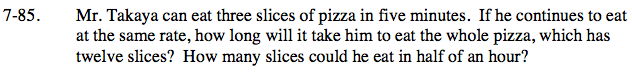Home > CC2 > Chapter 7 > Lesson 7.1.7 > Problem7-85

7-85.

Mr. Takaya can eat three slices of pizza in five minutes. If he continues to eat at the same rate, how long will it take him to eat the whole pizza, which has twelve slices? How many slices could he eat in half of an hour? Homework Help ✎$\text{If Mr. Takaya's rate is: }\frac{3\text{ slices}}{5\text{ minutes}}$

What can you multiply that by to get 12 slices?

$\frac{3\text{ slices}}{5 \text{ minutes}}\cdot \frac{4}{4}=\frac{12 \text{ slices}}{? \text{ minutes}}$

5 minutes (4) = 20 minutes
Mr. Takaya can eat the whole pizza in 20 minutes.

Try using this same method to find the number of slices Mr. Takaya can eat in half an hour (30 minutes).